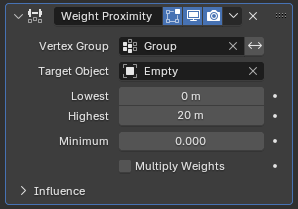# Vertex Weight Proximity Modifier

This modifier sets the weights of the given vertex group, based on the distance between the object (or its vertices), and another target object (or its geometry).

Warnung

This modifier does implicit clamping of weight values in the standard (0.0 to 1.0) range. All values below 0.0 will be set to 0.0, and all values above 1.0 will be set to 1.0.

## OptionsThe Vertex Weight Proximity modifier panel.
Vertex Group

The vertex group to affect.

Invert `<-->`

Inverts the influence of the selected vertex group. The setting reverses the weight values of the group.

Target Object

The object from which to compute distances.

Lowest

Distance mapping to 0.0 weight.

Highest

Distance mapping to 1.0 weight.

Minimum

Minimum value for vertex weight.

Multiply Weights

Multiply the calculated weights with the existing values in the vertex group.